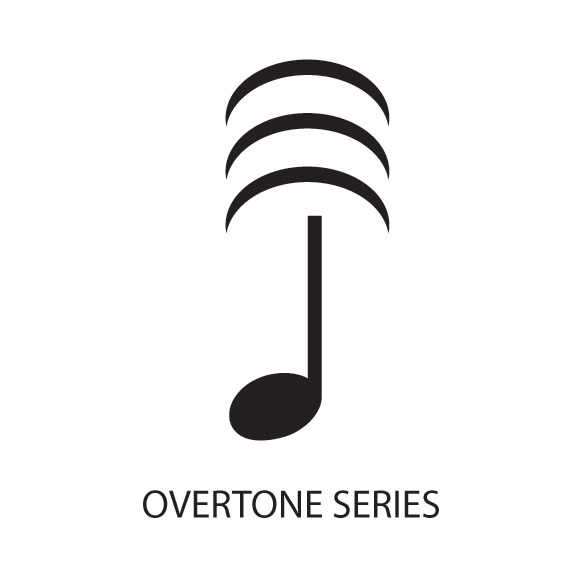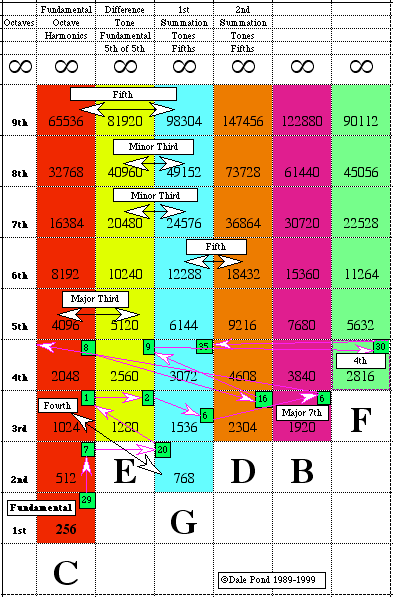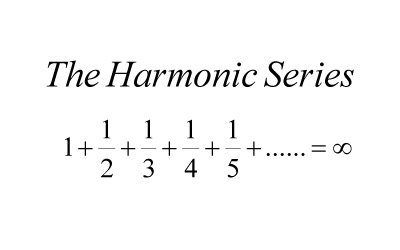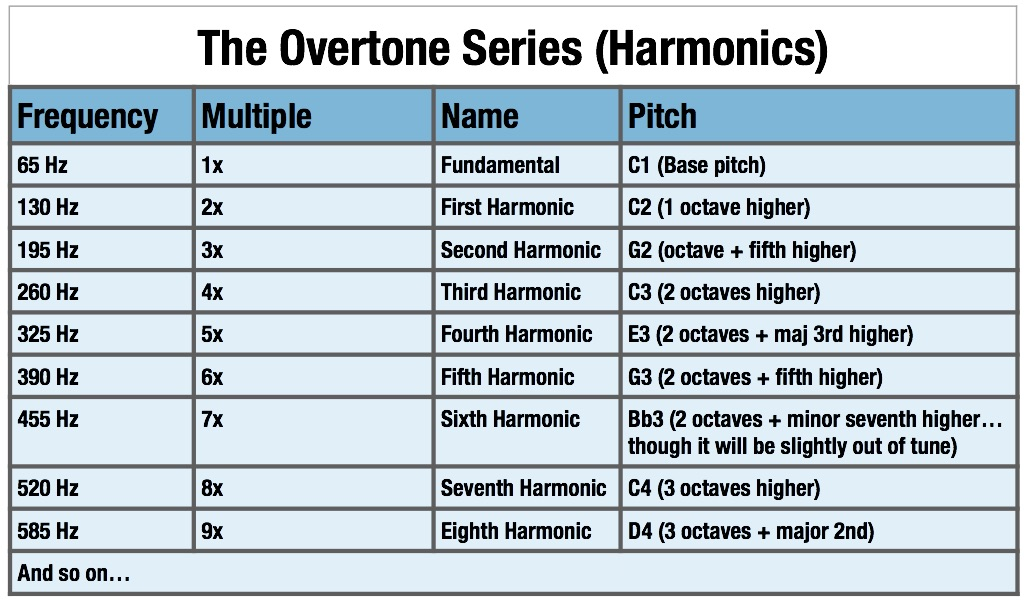# Overtone series

 Overtone SeriesOvertone Series Sympsionics Symbol

A naturally occurring series of overtones, partials or harmonics from a fundamental or any other tone, sound or frequency. The nature of this Progressive Evolution is discretely arithmetical according to what is vibrating whether it be a string, rod fastened at one end or both ends, diaphragm, volume of gas, etc. its substance, density, etc. as also by how the vibrating object is excited; striking, bowing or sympathetically. When as a volume the Progressive Evolution is geometric as in Russell's Scale of Locked Potentials.

Naturally Occurring Harmonics and Partials

Overtone Harmonics
(See MUTATIONS for more complete list.)
C:
First Octave
1 = c
Second Octave
2 = c'
3 = g'
Third Octave
4 = c''
5 = e''
6 = g''
7 = bb (it's actually about half way between a and bb)
Fourth Octave
8 = c'''
9 = d'''
10 = e'''
11 = f#''' (halfway between f and f#)
12 = g'''
13 = a'''
14 = bb''' (sort of)
15 = b'''
16 = c
17 = c#/db
18 = d
19 = eb (a bit flatter)
20 = e
21 = ???
22 = f# (sort of)
23 = f# (a bit sharper)
24 = g

This video contains an excellent description of overtones.Naturally Occurring Harmonics and Partials

Rod Fixed at Both Ends
"A rod fixed at both ends and caused to vibrate transversely divides itself in the same manner as a string vibrating transversely.

"But the succession of its overtones is not the same as those of a string, for while the series of tones emitted by the string is expressed by the natural numbers, 1, 2, 3, 4, 5, etc., the series of tones emitted by the rod is expressed by the squares of the odd numbers, 3, 5, 7, 9, etc." [from "Sound" by John Tyndall]

Rod Fixed at One End
"A rod fixed at one end can also vibrate as a whole, or can divide itself into vibrating segments separated from each other by nodes.

"In this case the rate of vibration of the fundamental tone is to that of the first overtone as 4:25, or as the square of 2 to the square of 5. From the first division onwards the rates of vibration are proportional to the squares of the odd numbers, 3, 5, 7, 9, etc.

"With rods of different lengths the rate of vibration is inversely proportional to the square of the length of the rod." [from "Sound", John Tyndall]Harmonic Series

1.20 - Evolution and Devolution of Frequency
1.23 - Power of Harmonics through Summation Tones
12.18 - Multiple Octave Progression
12.19 - Fibonacci Relationships
12.21 - Fibonacci Whole Numbers v Irrational Decimal near Equivalents
12.38 - Orbital revolution
14.15 - Movement Caused by Spirit
15.15 - Progressive Dissociation
15.15.05 - Progressive Association
3.04 - Power Accumulation via Fibonacci-like Patterns
8.17 - Law of Harmonic Vibrations
8.22 - Law of Harmonic Pitch
9.8 - Spontaneous Creation of Harmonic Series
9.9 - Sympathy or Harmony Between Harmonics or Overtones
arithmetic progression
arithmetical progression
Differentiation
Dissolution
Evolution
Fibonacci Relationships
Fibonacci Series
Overtones Developed Musically
Figure 8.5 - Summation Tones
Fractal
fugue
Genesis of the Scale
Geometrical Progression
Golden Section
Growth
harmonic progression
Harmonic Series
Harmonic
Interval
Law of Harmonic Pitch
Law of Harmonic Vibrations
Life
major
major key
major scale
Master Tone
master tones
Medu-Neter
Mid-tone
Motion
Movement
musical progression
Neter
octave tones
Overtone Position
Overtone
partial
PHI